Planet Quantum News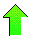Contents News View

 Jun 10, 2005 - A Generalization of Adamchik's Formulas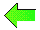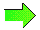This note (PDF version) discusses a generalization of some formulas in "A Class of Logarithmic Integrals" by Victor Adamchik.  Adamchik's paper proves 6 different general propositions allowing Adamchik to solve integrals such as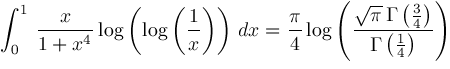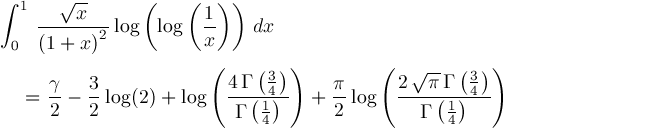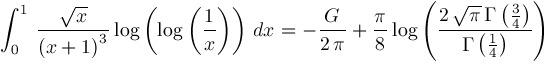Generally, I like Adamchik's paper.  The most interesting proposition is Proposition 1 which Adamchik attributes to G. Almkvist and A. Meurman.  The proofs for Adamchik's Proposition 3, Proposition 5, and Proposition 6 are more drawn out and tortured than necessary.  My theorem below is proved in less space and generalizes half the propositions in Adamchik's paper.

## Definition of Two Functions

Definition.For convenience, define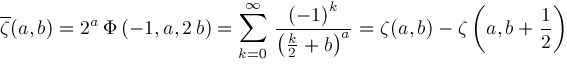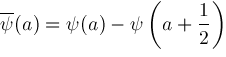Function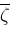has properties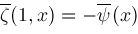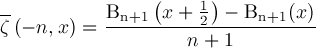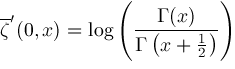The following theorem generalizes Adamchik's Proposition 3, Proposition 5, and Proposition 6.

## Generalization of Adamchik's Propositions 3, 5, and 6

Theorem.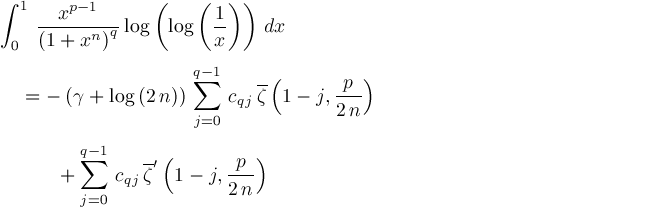where coefficients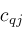are determined by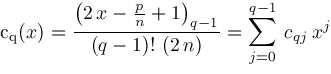Proof.Since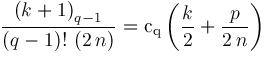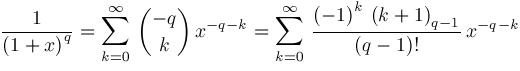We get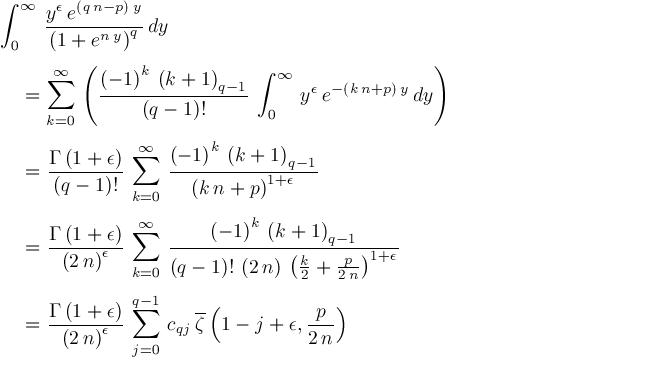Therefore,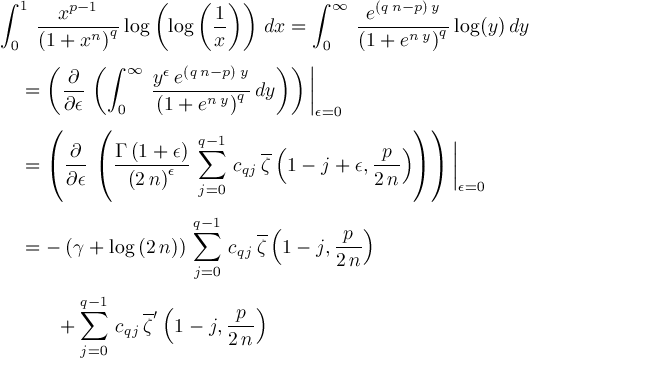Comment.The first few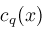are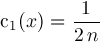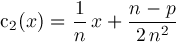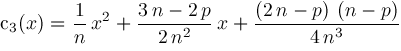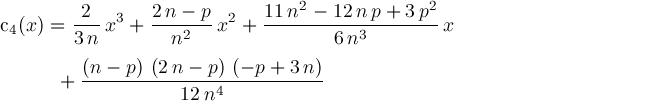The proof for Adamchik's Proposition 4 can also be simpler:

Theorem.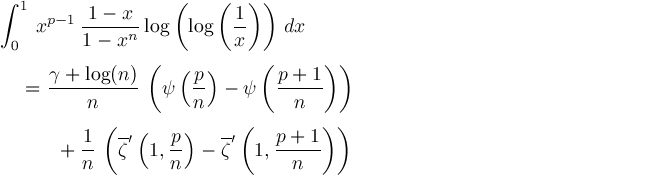Proof.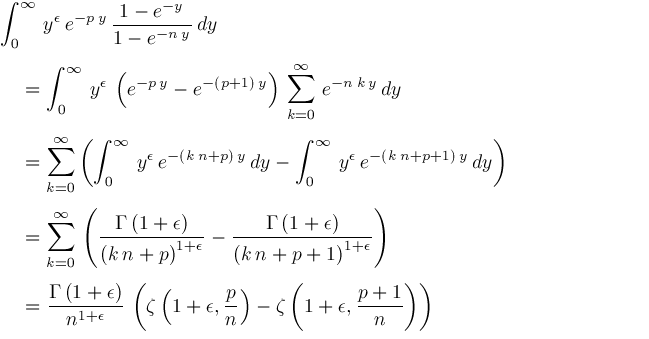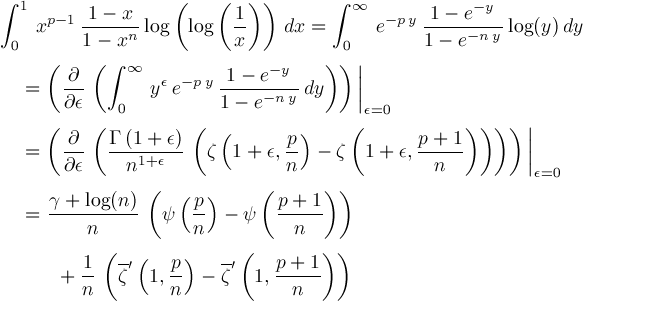## References

The first reference is Victor Adamchik's paper. The other
papers also discuss expression of definite integrals
in terms of derivatives of products of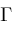and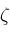functions.

Geddes, K. O. and Scott, T. C. (1989) "Recipes for Classes of Definite Integrals Involving Exponentials and Logarithms", Computers and Mathematics, Kaltofen, E. and Watt, S. M. (eds.), 192-201, Springer-Verlag.

Williams, Kenneth S. and Zhang, Nan Yue (1993) "Special Values of the Lerch Zeta Function and the Evaluation of Certain Integrals", Proc. Amer. Math. Soc., 119(1), 35-49.

Zhang, Nan Yue and Williams, Kenneth S. (1993) "Applications of the Hurwitz Zeta Function to the Evaluation of Certain Integrals", Canad. Math. Bull., 36(3), 373-384.